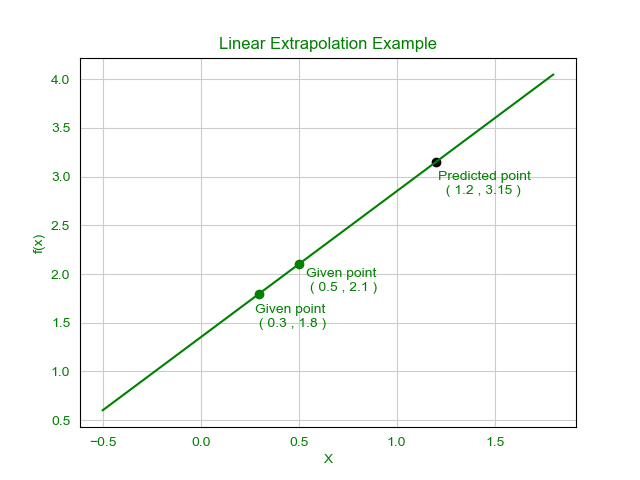# Program to implement Linear Extrapolation

What is Extrapolation?
Extrapolation is the process in mathematics where the required value is estimated beyond the range the of the given variable range. Extrapolation is often used to estimate the data of some observation below or above the given range. Extrapolation is also referred to as a mathematical prediction to predict values by observing the relationship between the given variables. There are many processes of Extrapolation.Here only Linear Extrapolation will be discussed. This process was first described by Thomas D. Clareson in 1959 in his book of science. He referred to it as a meaningful prediction by understanding the given data.

How to calculate Linear Exptrapolation?

The method is useful when the linear function is given. It is done by drawing a tangent and extending it beyond the limit. Linear Extrapolation gives a very good result when the point to be predicted is not very far from the rest of the points.

Extrapolation formula:Hereandare two given points and x is the point fow which we want to predict the value of y.

Examples:

Input:,, x = 1.2
Output: y = 3.15Implementation:

## C++

 // C++ code for the implementation   // of Linear extrapolation     #include  using namespace std;     // Consider a structure  // to keep each pair of x and y together  struct Data {      double x, y;  };     // Function to calculate  // the linear extrapolation  double extrapolate(Data d[], double x)  {      double y;      y = d.y          + (x - d.x)                / (d.x - d.x)                * (d.y - d.y);         return y;  }     // Driver Code  int main()  {      // Sample dataset      Data d[] = { { 1.2, 2.7 }, { 1.4, 3.1 } };         // Sample x value      double x = 2.1;         // Finding the extrapolation      cout << "Value of y at x = 2.1 : "          << extrapolate(d, x);         return 0;  }

## Java

 // Java code for the implementation of  // Linear extrapolation   class GFG  {         // Function to calculate the linear  // extrapolation   static double extrapolate(double[][] d, double x)   {       double y = d + (x - d) /                   (d - d) *                   (d - d);          return y;   }      // Driver Code   public static void main (String[] args)  {         // Sample dataset   double[][] d = {{ 1.2, 2.7 },{ 1.4, 3.1 }};      // Sample x value   double x = 2.1;      // Finding the extrapolation   System.out.println("Value of y at x = 2.1 : " +                      extrapolate(d, x));   }  }     // This code is contributed by chandan_jnu

## Python3

 # Python3 code for the implementation of  # Linear extrapolation      # Function to calculate the linear  # extrapolation   def extrapolate(d, x):      y = (d + (x - d) /          (d - d) *          (d - d));          return y;      # Driver Code      # Sample dataset   d = [[ 1.2, 2.7 ], [1.4, 3.1 ]];      # Sample x value   x = 2.1;      # Finding the extrapolation   print("Value of y at x = 2.1 :",                extrapolate(d, x));      # This code is contributed by mits

## C#

 // C# code for the implementation of  // Linear extrapolation   class GFG  {         // Function to calculate the linear  // extrapolation   static double extrapolate(double[,] d, double x)   {       double y = d[0,1] + (x - d[0,0]) /                   (d[1,0] - d[0,0]) *                   (d[1,1] - d[0,1]);          return y;   }      // Driver Code   static void Main()  {         // Sample dataset   double[,] d = {{ 1.2, 2.7 },{ 1.4, 3.1 }};      // Sample x value   double x = 2.1;      // Finding the extrapolation   System.Console.WriteLine("Value of y at x = 2.1 : " +                      extrapolate(d, x));   }  }     // This code is contributed by chandan_jnu

## PHP

 

Output:

Value of y at x = 2.1 : 4.5


My Personal Notes arrow_drop_upCheck out this Author's contributed articles.

If you like GeeksforGeeks and would like to contribute, you can also write an article using contribute.geeksforgeeks.org or mail your article to contribute@geeksforgeeks.org. See your article appearing on the GeeksforGeeks main page and help other Geeks.

Please Improve this article if you find anything incorrect by clicking on the "Improve Article" button below.